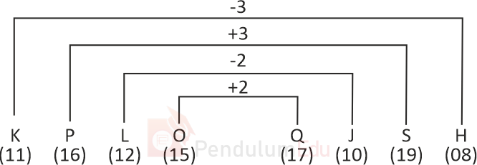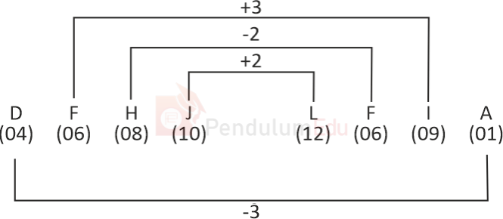Question of The Day10-03-2022

Select the option that has "related four letter clusters" in the same way as the below cluster.

KPLO: QJSH

Correct Answer : a ) DFHJ: LFIA

Explanation :

Let us assume A is numbered as 1, B as 2, and so on till Z is numbered as 26

The common logic applied here is

KPLO: QJSHSimilarly, if we check in all options, only in option A, the same pattern is followed.

DFHJ: LFIAHence, (a) is the correct answer.0